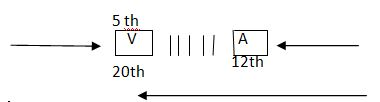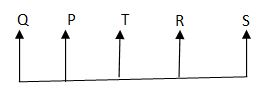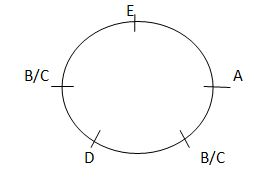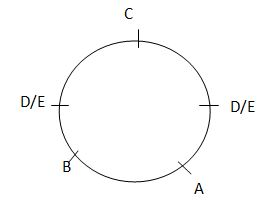# Data Sufficiency Practice set 4

Directions (1-10): Each of the question below consists of a question and two statements numbered I and II given below it. You have to decide whether the data provided in the statements are sufficient t answer the question.

1) What is Sunita`s rank form the top in the class of 40students?

(i) Rani is 4 ranks below Sunita and isthirty first from the bottom.

(ii) Amit is two ranks above Sunita and is thirty seventh from the bottom.

a) If the data in statements I alone are sufficient to answer the question, while the data in statement II alone are not sufficient to answer the question.

b) If the data in statements II alone are sufficient to answer the question, while the data in statement I alone are not sufficient to answer the question.

c) If the data in statements I alone or in statement II alone are sufficient to answer the question.

d) If the data in both the statement I and II are not sufficient to answer the question.

e) If the data in both the statements I and II together are necessary to answer the question.

1.(c)

From statement I

Rank of Rani from the top = 40  – 31 + 1 = 10

Therefore, rank of Sunita = 10 – 4 = 6th

From statement II

Rank of Amit from the top = 40 – 37 + 1 = 4th

Therefore, rank of Sunita = 4 +2 = 6th

2)What is the code for `Comfort` in the code language?

(i) `pa k eta` means `sit with comfort`.

(ii) `Ke da raj a` means `she sat with confidence`.

a) If the data in statements I alone are sufficient to answer the question, while the data in statement II alone are not sufficient to answer the question.

b) If the data in statements II alone are sufficient to answer the question, while the data in statement I alone are not sufficient to answer the question.

c) If the data in statements I alone or in statement II alone are sufficient to answer the question.

d) If the data in both the statement I and II are not sufficient to answer the question.

e) If the data in both the statements I and II together are necessary to answer the question.

2.(d)

From both the statements

Pa   ke  ta → sit with comfort

Ke da ra  ja → she sat with confidence

Code for `comfort` is either `pa` or `ta`

3)How is Nisha related to Nidhi?

(i) Nisha`s mother is sister of Nidhi`s father.

(ii) Nidhi is the daughter of Nisha`s grand – father`s only son.

a) If the data in statements I alone are sufficient to answer the question, while the data in statement II alone are not sufficient to answer the question.

b) If the data in statements II alone are sufficient to answer the question, while the data in statement I alone are not sufficient to answer the question.

c) If the data in statements I alone or in statement II alone are sufficient to answer the question.

d) If the data in both the statement I and II are not sufficient to answer the question.

e) If the data in both the statements I and II together are necessary to answer the question.

(a)   From statement I

Nisha is cousin of Nidhi.

From statement II

Nisha is cousin or brother / sister of Nidhi.

4)Kumud is facing which direction?

(i) Nikhil is facing North and if he turns to his left he faces opposite direction as that of kumud.

(ii) Saroj who is facing East is to the right of kumud.

a) If the data in statements I alone are sufficient to answer the question, while the data in statement II alone are not sufficient to answer the question.

b) If the data in statements II alone are sufficient to answer the question, while the data in statement I alone are not sufficient to answer the question.

c) If the data in statements I alone or in statement II alone are sufficient to answer the question.

d) If the data in both the statement I and II are not sufficient to answer the question.

e) If the data in both the statements I and II together are necessary to answer the question.

(a)

From statement I

Nikhil after turning to his left would face towards west.

Therefore, kumud is facing towards east.

From statement II

It is not possible to determine kumud is facing which direction.5)

5) What is the colour of the fresh grass?

(i) Blue is called green, red is called orange. Orange is called yellow.

(ii) Yellow is called white, white is called black, green is called brown and brown is called purple.

a) If the data in statements I alone are sufficient to answer the question, while the data in statement II alone are not sufficient to answer the question.

b) If the data in statements II alone are sufficient to answer the question, while the data in statement I alone are not sufficient to answer the question.

c) If the data in statements I alone or in statement II alone are sufficient to answer the question.

d) If the data in both the statement I and II are not sufficient to answer the question.

e) If the data in both the statements I and II together are necessary to answer the question.

(e)

From both the statements

The colour of fresh grass is green.

Here green is called brown.

6)Who is tallest among the five boys Samar, Ramesh, Rakesh, Karan and Vishwas?

(i) Rakesh is taller than karan and Ramesh, but not as tall as Samar.

(ii) Samar is not the tallest.

a) If the data in statements I alone are sufficient to answer the question, while the data in statement II alone are not sufficient to answer the question.

b) If the data in statements II alone are sufficient to answer the question, while the data in statement I alone are not sufficient to answer the question.

c) If the data in statements I alone or in statement II alone are sufficient to answer the question.

d) If the data in both the statement I and II are not sufficient to answer the question.

e) If the data in both the statements I and II together are necessary to answer the question.

6.(e)

From both the statements

Vishwas is the tallest.

7)How many children are there in the row of children facing North?

(i) Vasudha who is fifth from the left end is eighth to the left of Anisha who is twelfth from the right end.

(ii) Rahul is fifth to the left of Neeta who is seventh from the right end and eighteenth from the left end.

a) If the data in statements I alone are sufficient to answer the question, while the data in statement II alone are not sufficient to answer the question.

b) If the data in statements II alone are sufficient to answer the question, while the data in statement I alone are not sufficient to answer the question.

c) If the data in statements I alone or in statement II alone are sufficient to answer the question.

d) If the data in both the statement I and II are not sufficient to answer the question.

e) If the data in both the statements I and II together are necessary to answer the question.

(c)

From statement ITotal number of children = 20 + 5 -1 = 24

From statement II

Total number of children = 7 + 18 – 1 = 24

8)What is the value of 144 \$ 16* 7 # 9 ?

(i) \$ means `÷`,`*` means `×` and `#` means `÷`.

(ii) 16 \$ 4 * 2 # 2 = 10

a) If the data in statements I alone are sufficient to answer the question, while the data in statement II alone are not sufficient to answer the question.

b) If the data in statements II alone are sufficient to answer the question, while the data in statement I alone are not sufficient to answer the question.

c) If the data in statements I alone or in statement II alone are sufficient to answer the question.

d) If the data in both the statement I and II are not sufficient to answer the question.

e) If the data in both the statements I and II together are necessary to answer the question.

8.(c)

From statement I

144 \$ 16 * 7 # 9

Or, ? = 144 ÷ 16 × 7 + 9

Or, ? = 9 × 7 + 9

Or, ? = 63 + 9 = 72

From statement II

16 \$ 4 * 2 # 2 = 10

OR, 16 ÷ 4 × 2 + 2 = 10

OR, 4 × 2 + 2 = 10

Now, we can solve the question.

9)Among P, Q, R, S, and T, sitting in a straight line, facing North, who sits exactly in the middle of the line ?

(i) P sits third to left of S. T is an immediate neighbour of P as well as R.

(ii) T sits second to left of S. Q is not an immediate neighbour of either T or S.

a) If the data in statements I alone are sufficient to answer the question, while the data in statement II alone are not sufficient to answer the question.

b) If the data in statements II alone are sufficient to answer the question, while the data in statement I alone are not sufficient to answer the question.

c) If the data in statements I alone or in statement II alone are sufficient to answer the question.

d) If the data in both the statement I and II are not sufficient to answer the question.

e) If the data in both the statements I and II together are necessary to answer the question.

9. (e)   From both the statements

T sits exactly in the middle of the line.10) Among five friends A, B, C D and E, sitting around a circular table, facing the centre, who sits to the immediate right of A?

(i) E sits third to right of D. A is not an immediate neighbour of D.

(ii) C sits second to left of B. A is not an immediate neighbour of C.

a) If the data in statements I alone are sufficient to answer the question, while the data in statement II alone are not sufficient to answer the question.

b) If the data in statements II alone are sufficient to answer the question, while the data in statement I alone are not sufficient to answer the question.

c) If the data in statements I alone or in statement II alone are sufficient to answer the question.

d) If the data in both the statement I and II are not sufficient to answer the question.

e) If the data in both the statements I and II together are necessary to answer the question.

10.(a)

From statement I

E sits to the immediate right of A.

From statement II